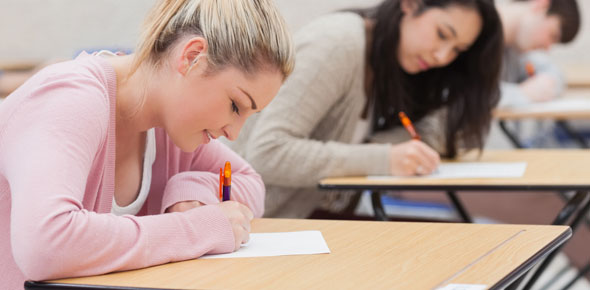# Practice Paper For

19 Questions | Total Attempts: 23Settings.

Related Topics
• 1.
60+190=
• A.

245

• B.

250

• C.

255

• 2.
12x12=
• A.

122

• B.

144

• C.

160

• 3.
608 sweets shared among two people=
• A.

304

• B.

290

• C.

208

• 4.
What is 2.2+2.0=
• A.

4.4

• B.

4.2

• C.

4.6

• 5.
What is higher  1/2 or 1/4 or 1/8
• A.

1/8

• B.

1/4

• C.

1/2

• 6.
There are .................. centimeres in a metre?
• 7.
There are ................ centimetres in an inch
• 8.
These are test results20   33   34  98   100   What is the highest test score?
• 9.
What is 50% of £ 3.00
• A.

£1.50

• B.

£1.00

• C.

£1.90

• 10.
What name is given to an angle over 90 degrees
• A.

Obtuse

• B.

Right

• C.

Acute

• 11.
What is the name given to an angle below 90 degrees
• A.

Obtuse

• B.

Acute

• C.

Right

• 12.
Put 205 pence into pounds
• 13.
Put this sequence in order from highest to lowest  90,100,110,55,155
• 14.
What is 1/2 of 20
• 15.
What is the peimeter of a triangle with equal sides of 30 cm
• A.

90cm

• B.

100cm

• C.

110cm

• 16.
What is an 2.2 times 2
• 17.
What shape has five sides?
• A.

Pentagon

• B.

Hexagon

• C.

Decahdron

• 18.
22.2 + 2.6=
• A.

24.8

• B.

25.8

• C.

26.8

• 19.
What is half of 100 multiplied by 5